#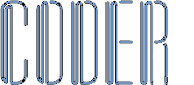OOP - Transformations

## Overview

Mathematical transformations are usually drawn on hard copy graph paper. This application demonstrates simple transformations. There are four types of transformation shown here, which are Enlargement, which can be positive or negative, Reflection, with a variable line of symmetry, Rotation with a variable centre of rotation and positive and negative angles of rotation, and the fourth transformation is Translation. These are the type of transformations that you're often asked to draw in maths exams...

## The Form

This is a basic Windows Form containing just a MenuStrip, and four extended Panels, one for each type of transformation. The Form hosts the event handlers for the MouseMove and MouseClick events for all four of the Panels. It also hosts the event handlers for the ValueChanged and KeyPress events for all of the NumericUpDown controls used throughout the application.

The handlers are wired up with AddHandler statements:

```AddHandler Enlargement1.MouseMove, AddressOf panels_MouseMove
```

These are the handlers panels_MouseMove and panels_MouseClick. These two handlers record the user mouse input used in drawing a base shape through clicking on the grid vertices in the graph image...

```Private Sub panels_MouseMove(ByVal sender As Object, ByVal e As System.Windows.Forms.MouseEventArgs)
Dim index As Integer = Array.IndexOf(panels, sender)

Dim r As Integer = -1
Dim c As Integer = -1

For x As Integer = 0 To 16
If e.Y >= rowY(x) - 3 AndAlso e.Y <= rowY(x) + 3 Then
r = rowY(x)
Exit For
End If
Next
For x As Integer = 0 To 16
If e.X >= columnX(x) - 3 AndAlso e.X <= columnX(x) + 3 Then
c = columnX(x)
Exit For
End If
Next
If c > -1 AndAlso r > -1 Then
sharedVariables.allHighlights(index) = New Point(c, r)
Else
sharedVariables.allHighlights(index) = Nothing
End If
DirectCast(sender, Panel).Refresh()
End Sub

Private Sub panels_MouseClick(ByVal sender As Object, ByVal e As System.Windows.Forms.MouseEventArgs)
Dim index As Integer = Array.IndexOf(panels, sender)
If sharedVariables.allHighlights(index) <> Nothing Then
If sharedVariables.allVertices(index).Contains(sharedVariables.allHighlights(index)) Then
sharedVariables.allVertices(index).Remove(sharedVariables.allHighlights(index))
Else
End If
DirectCast(sender, Panel).Refresh()
End If
End Sub
```

The other events handled in the Form code are the ValueChanged and the KeyPress events for all of the NumericUpDown controls used in the application...

```AddHandler Enlargement1.nudXPosition.ValueChanged, AddressOf numericupdowns_ValueChanged

```

These are the event handlers for those controls...

The calculations for the transformed shapes are all done in the Panel's paint event. Changing any input just causes the Panel at the top of the z-order to repaint. All of the controls used are primarily value changers for the graphical output.

The KeyPress event is used to restrict user input to just mouse input.

```Private Sub numericupdowns_ValueChanged(ByVal sender As System.Object, ByVal e As System.EventArgs)
Enlargement1.Refresh()
Reflection1.Refresh()
Rotation1.Refresh()
Translation1.Refresh()
End Sub

Private Sub numericupdowns_KeyPress(ByVal sender As System.Object, ByVal e As System.Windows.Forms.KeyPressEventArgs)
e.Handled = True
End Sub
```

## The Panels

Three of the Panels have a cross indicator which shows the centre of the transformation, or the offset applied in the transformation. The remaining Panel has a line of symmetry. These serve as a visual representation of the variables used in the transformation

```Public Shared Sub drawCross(ByVal g As Graphics, ByVal l As Integer, ByVal t As Integer)
Dim p As New Pen(Color.LimeGreen, 2)
g.DrawLine(p, l, t - 10, l, t + 10)
g.DrawLine(p, l - 10, t, l + 10, t)
End Sub
```

```Public Shared Sub drawMirrorLine(ByVal g As Graphics, ByVal l As Integer, ByVal t As Integer, ByVal v As Integer, ByVal x As Integer)
Dim p As New Pen(Color.LimeGreen, 2)
p.DashStyle = Drawing2D.DashStyle.Dash

Dim diagonals() As Integer = {5, 4, 3, 2, 1, 0, 1, 2, 3, 4, 5}

Dim x1 As Integer
Dim y1 As Integer
Dim x2 As Integer
Dim y2 As Integer

Select Case x
Case 0 'Vertical
g.drawLine(p, l, 25, l, 425)
Case 1 'Horizontal
g.drawLine(p, 25, t, 425, t)
Case 2 'Diagonal - SW to NE
If v <= 2 Then
x1 = 25 + diagonals(v + 3) * 25
y1 = 425
x2 = 425
y2 = 25 + diagonals(v + 3) * 25
Else
x1 = 25
y1 = 425 - diagonals(v + 3) * 25
x2 = 425 - diagonals(v + 3) * 25
y2 = 25
End If
g.drawLine(p, x1, y1, x2, y2)
Case 3 'Diagonal - NW to SE
If v <= 2 Then
x1 = 25
y1 = 25 + diagonals(v + 3) * 25
x2 = 25 + (16 - diagonals(v + 3)) * 25
y2 = 425
Else
x1 = 25 + diagonals(v + 3) * 25
y1 = 25
x2 = 425
y2 = 25 + (16 - diagonals(v + 3)) * 25
End If
g.drawLine(p, x1, y1, x2, y2)
End Select

End Sub
```

### Enlargement Panel

The first of the extended Panels is the Enlargement Panel...

As you can see, mouse hovering over a grid vertex highlights that vertex.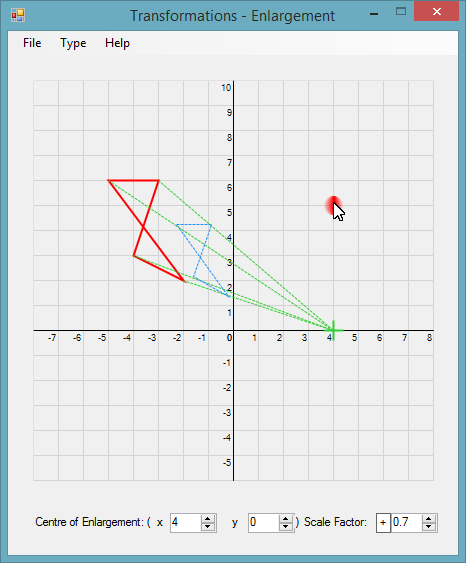The code for the Enlargement Panel is fairly simple.

The overridden Paint method is the coordinating procedure for all of the various drawing methods used.

```Protected Overrides Sub OnPaint(ByVal e As System.Windows.Forms.PaintEventArgs)
e.Graphics.SmoothingMode = Drawing2D.SmoothingMode.HighQuality

methods.drawGrid(e.Graphics, Me.Font)

Dim l As Integer = pointMethods.c2X(CInt(nudXPosition.Value))
Dim t As Integer = pointMethods.r2Y(CInt(nudYPosition.Value))
Dim s As Decimal = nudScale.Value

methods.drawShape(e.Graphics, sharedVariables.allVertices(0))
methods.drawEnlargedShape(e.Graphics, l, t, s, lblPolarity.Text, sharedVariables.allVertices(0))
methods.drawBorders(e.Graphics, Me.BackColor)
methods.drawCross(e.Graphics, l, t)
methods.drawHighlight(e.Graphics, sharedVariables.allHighlights(0))

MyBase.OnPaint(e)
End Sub
```

There are two other handlers contained in the Enlargement Panel code...

One is for a context menu providing a simple way to clear a drawing. The other is the Click handler for the positive/negative Label. Clicking the Label changes the text of the Label, and causes a repaint.

```Private Sub ClearToolStripMenuItem_Click(ByVal sender As System.Object, ByVal e As System.EventArgs) Handles ClearToolStripMenuItem.Click
sharedVariables.allVertices(0).Clear()
Me.Refresh()
End Sub

Private Sub lblPolarity_Click(ByVal sender As System.Object, ByVal e As System.EventArgs) Handles lblPolarity.Click
If lblPolarity.Text = "+" Then lblPolarity.Text = "-" Else lblPolarity.Text = "+"
Me.Refresh()
End Sub
```

### Reflection Panel

This is how the Reflection Panel appears at run time...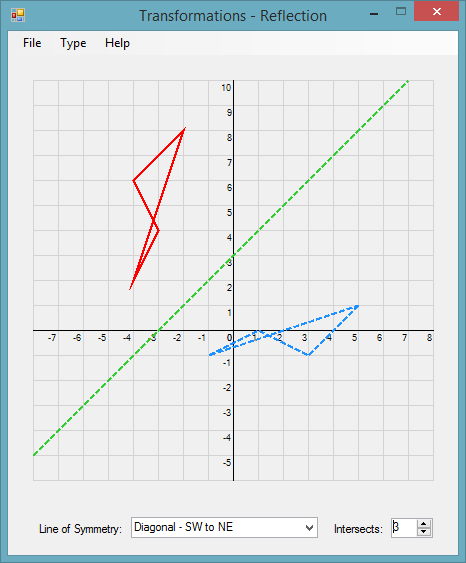The overridden Paint event is again kept very simple, calling a series of helper methods, each performing a single task...

```Protected Overrides Sub OnPaint(ByVal e As System.Windows.Forms.PaintEventArgs)

methods.drawGrid(e.Graphics, Me.Font)

Dim l As Integer = 25 + ((CInt(nudIntersection.Value) + 8) * 25)
Dim t As Integer = 25 + (Math.Abs(CInt(nudIntersection.Value) - 10) * 25)
Dim v As Integer = CInt(nudIntersection.Value)

methods.drawMirrorLine(e.Graphics, l, t, v, cboLine.SelectedIndex)
methods.drawShape(e.Graphics, sharedVariables.allVertices(1))
methods.drawMirrorShape(e.Graphics, sharedVariables.allVertices(1), v, cboLine.SelectedIndex)
methods.drawBorders(e.Graphics, Me.BackColor)
methods.drawHighlight(e.Graphics, sharedVariables.allHighlights(1))

MyBase.OnPaint(e)
End Sub
```

There are two other events handled, which are local to the Reflection Panel.

The first is a context menu click handler. All of the Panels have an encapsulated context menu.

The second handler is for the ComboBox used in choosing where to place the line of symmetry.

```Private Sub ClearToolStripMenuItem_Click(ByVal sender As System.Object, ByVal e As System.EventArgs) Handles ClearToolStripMenuItem.Click
sharedVariables.allVertices(1).Clear()
Me.Refresh()
End Sub

Private Sub cboLine_SelectedIndexChanged(ByVal sender As System.Object, ByVal e As System.EventArgs) Handles cboLine.SelectedIndexChanged
nudIntersection.Value = 0
nudIntersection.Minimum = -3
Me.Refresh()
End Sub
```

### Rotation Panel

This is how the Rotation Panel appears at run time...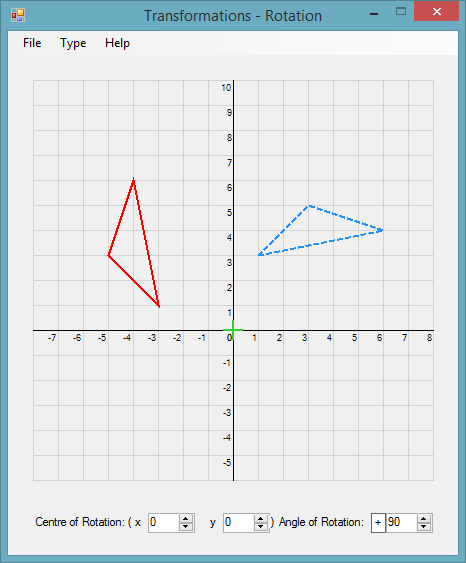Continuing the application-wide theme, the Rotation Panel Paint event is neat and kept simple...

```Protected Overrides Sub OnPaint(ByVal e As System.Windows.Forms.PaintEventArgs)

methods.drawGrid(e.Graphics, Me.Font)

Dim l As Integer = 25 + ((CInt(nudXCentre.Value) + 8) * 25)
Dim t As Integer = 25 + (Math.Abs(CInt(nudYCentre.Value) - 10) * 25)
Dim v As Integer = CInt(nudAngle.Value)

methods.drawShape(e.Graphics, sharedVariables.allVertices(2))
methods.drawRotatedShape(e.Graphics, sharedVariables.allVertices(2), v, l, t, lblPolarity.Text)
methods.drawBorders(e.Graphics, Me.BackColor)
methods.drawCross(e.Graphics, l, t)
methods.drawHighlight(e.Graphics, sharedVariables.allHighlights(2))

MyBase.OnPaint(e)
End Sub
```

This Panel also has a context menu and a polarity Label...

```Private Sub lblPolarity_Click(ByVal sender As System.Object, ByVal e As System.EventArgs) Handles lblPolarity.Click
If lblPolarity.Text = "-" Then lblPolarity.Text = "+" Else lblPolarity.Text = "-"
Me.Refresh()
End Sub

Private Sub ClearToolStripMenuItem_Click(ByVal sender As System.Object, ByVal e As System.EventArgs) Handles ClearToolStripMenuItem.Click
sharedVariables.allVertices(2).Clear()
Me.Refresh()
End Sub
```

### Translation Panel

This is how the fourth Panel appears at runtime...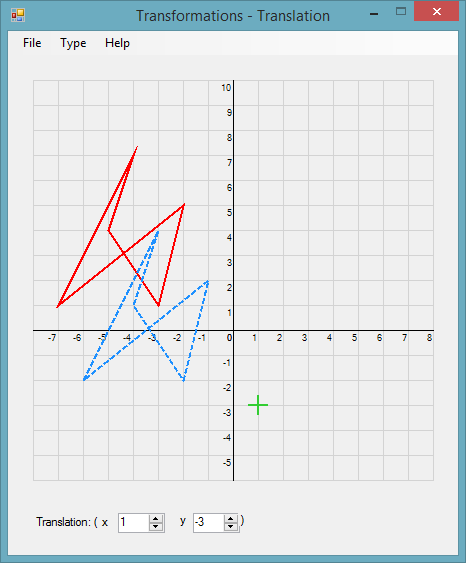Again the code is concise and easy to read...

```Protected Overrides Sub OnPaint(ByVal e As System.Windows.Forms.PaintEventArgs)

methods.drawGrid(e.Graphics, Me.Font)

Dim l As Integer = 25 + ((CInt(nudXPosition.Value) + 8) * 25)
Dim t As Integer = 25 + (Math.Abs(CInt(nudYPosition.Value) - 10) * 25)

methods.drawShape(e.Graphics, sharedVariables.allVertices(3))
methods.drawTranslatedShape(e.Graphics, sharedVariables.allVertices(3), l, t)
methods.drawBorders(e.Graphics, Me.BackColor)
methods.drawCross(e.Graphics, l, t)
methods.drawHighlight(e.Graphics, sharedVariables.allHighlights(3))

MyBase.OnPaint(e)
End Sub
```

The Translation Panel, as all the other Panels, has a context menu

```Private Sub ClearToolStripMenuItem_Click(ByVal sender As System.Object, ByVal e As System.EventArgs) Handles ClearToolStripMenuItem.Click
sharedVariables.allVertices(3).Clear()
Me.Refresh()
End Sub
```

## The Drawing Methods

drawGrid is common to all of the Panels. It draws the 'Graph' grid and labels.

```Public Shared Sub drawGrid(ByVal g As Graphics, ByVal f As Font)
g.DrawRectangle(Pens.LightGray, New Rectangle(25, 25, 400, 400))

For c As Integer = 50 To 400 Step 25
g.DrawLine(Pens.LightGray, c, 25, c, 425)
Next
For r As Integer = 50 To 400 Step 25
g.DrawLine(Pens.LightGray, 25, r, 425, r)
Next

g.DrawLine(Pens.Black, 225, 25, 225, 425)
g.DrawLine(Pens.Black, 25, 275, 425, 275)

Dim lblWidth As Integer

For x As Integer = -8 To 8
lblWidth = CInt(g.MeasureString(x.ToString, New Font(f.FontFamily, 7)).Width)
g.DrawString(x.ToString, New Font(f.FontFamily, 7), Brushes.Black, 25 + ((x + 8) * 25) - lblWidth, 276)
Next

For y As Integer = -6 To 10
lblWidth = CInt(g.MeasureString(y.ToString, New Font(f.FontFamily, 7)).Width)
g.DrawString(y.ToString, New Font(f.FontFamily, 7), Brushes.Black, 225 - lblWidth, 426 - (y + 6) * 25)
Next
End Sub
```

drawShape is common to all of the Panels. The main difference in the four Panels' Paint event is one unique drawing method for each Panel...

```Public Shared Sub drawShape(ByVal g As Graphics, ByVal vertices As List(Of Point))
If vertices.Count > 2 Then
g.DrawPolygon(New Pen(Color.Red, 2), vertices.ToArray)
Else
For x As Integer = 0 To vertices.Count - 1
g.FillEllipse(Brushes.Red, New Rectangle(vertices(x).X - 3, vertices(x).Y - 3, 6, 6))
Next
End If
End Sub
```

## These are the four unique drawing methods

### drawEnlargedShape

This contains all of the calculations used in rendering an enlarged shape...

```Public Shared Sub drawEnlargedShape(ByVal g As Graphics, ByVal l As Integer, ByVal t As Integer, ByVal s As Decimal, ByVal polarity As String, ByVal vertices As List(Of Point))
If vertices.Count >= 3 Then
Dim pen As New Pen(Color.LimeGreen)
pen.DashStyle = Drawing2D.DashStyle.Dash

Dim newPoints As New List(Of Point)()

For x As Integer = 0 To vertices.Count - 1
Dim distance As Single = measurement.lineLength(New Point(l, t), vertices(x))
Dim angle As Double = (If(polarity.Equals("+"), 360, 180)) - angles.FindAngle(New Point(l, t), vertices(x))

Dim scaleDistance As Decimal = CDec(distance * s)

'Turn degrees to radians (because of the sin and cos operations)
Dim angleRadians As Double = angle * (Math.PI / 180)

'Calculate X1 And Y1
Dim pointX1 As Integer = CInt(l + Math.Cos(angleRadians) * scaleDistance)
Dim pointY1 As Integer = CInt(t - Math.Sin(angleRadians) * scaleDistance)

Next

If polarity.Equals("+") Then
For x As Integer = 0 To vertices.Count - 1
g.DrawLine(pen, l, t, If(s > 1D, newPoints(x).X, vertices(x).X), If(s > 1D, newPoints(x).Y, vertices(x).Y))
Next
Else
For x As Integer = 0 To vertices.Count - 1
g.DrawLine(pen, l, t, vertices(x).X, vertices(x).Y)
g.DrawLine(pen, l, t, newPoints(x).X, newPoints(x).Y)
Next
End If

Dim pen2 As New Pen(Color.DodgerBlue)
pen2.DashStyle = Drawing2D.DashStyle.Dash

g.DrawPolygon(pen2, newPoints.ToArray)
End If
End Sub
```

### drawMirrorShape

This again contains all of the calculations needed for the specified transformation...

```Public Shared Sub drawMirrorShape(ByVal g As Graphics, ByVal vertices As List(Of Point), ByVal v As Integer, ByVal x As Integer)
If vertices.Count < 3 Then
Return
End If

Dim pen As New Pen(Color.DodgerBlue, 2)
pen.DashStyle = Drawing2D.DashStyle.Dash

Dim gridPoints As New List(Of Point)
For Each p As Point In vertices
Next p

Dim reflectionPoints As New List(Of Point)
Select Case x
Case 0 'Vertical
For Each p As Point In gridPoints
reflectionPoints.Add(New Point(pointMethods.swapPolarity(p.X - v) + v, p.Y))
Next p
Case 1 'Horizontal
For Each p As Point In gridPoints
reflectionPoints.Add(New Point(p.X, pointMethods.swapPolarity(p.Y - v) + v))
Next p
Case 2 'Diagonal - SW to NE
For Each p As Point In gridPoints
reflectionPoints.Add(New Point(p.Y - v, p.X + v))
Next p
Case 3 'Diagonal - NW to SE
For Each p As Point In gridPoints
reflectionPoints.Add(New Point(pointMethods.swapPolarity(p.Y - v), pointMethods.swapPolarity(p.X) + v))
Next p
End Select

Dim reflectionCoordinates As New List(Of Point)
For Each p As Point In reflectionPoints
Next

g.DrawPolygon(pen, reflectionCoordinates.ToArray)

End Sub
```

### drawRotatedShape

This contains all of the calculations necessary to draw a rotation transformation...

```Public Shared Sub drawRotatedShape(ByVal g As Graphics, ByVal vertices As List(Of Point), ByVal r As Integer, ByVal l As Integer, ByVal t As Integer, ByVal polarity As String)
If vertices.Count < 3 Then
Return
End If

Dim pen As New Pen(Color.DodgerBlue, 2)
pen.DashStyle = Drawing2D.DashStyle.Dash

Dim gridPoints As New List(Of Point)
For Each p As Point In vertices
Next p

l = pointMethods.X2c(l)
t = pointMethods.Y2r(t)

Dim rotationPoints As New List(Of Point)

Select Case polarity & r.ToString()
Case "+90", "-270"
For Each p As Point In gridPoints
rotationPoints.Add(New Point((p.Y - t) + l, -(p.X - l) + t))
Next p
Case "-180", "+180"
For Each p As Point In gridPoints
rotationPoints.Add(New Point(-(p.X - l) + l, -(p.Y - t) + t))
Next p
Case "+270", "-90"
For Each p As Point In gridPoints
rotationPoints.Add(New Point(-(p.Y - t) + l, p.X - l + t))
Next p
Case Else
For Each p As Point In gridPoints
Next p
End Select

Dim rotationCoordinates As New List(Of Point)
For Each p As Point In rotationPoints
Next

g.DrawPolygon(pen, rotationCoordinates.ToArray)

End Sub
```

### drawTranslatedShape

This is the simplest of the transformations. A translated shape is simply offset from the original shape...

```Public Shared Sub drawTranslatedShape(ByVal g As Graphics, ByVal vertices As List(Of Point), ByVal l As Integer, ByVal t As Integer)
If vertices.Count < 3 Then
Return
End If

Dim pen As New Pen(Color.DodgerBlue, 2)
pen.DashStyle = Drawing2D.DashStyle.Dash

Dim gridPoints As New List(Of Point)
For Each p As Point In vertices
Next p

l = pointMethods.X2c(l)
t = pointMethods.Y2r(t)

Dim translationPoints As New List(Of Point)

For Each p As Point In gridPoints
translationPoints.Add(New Point(p.X + l, p.Y + t))
Next p

Dim translationCoordinates As New List(Of Point)
For Each p As Point In translationPoints
Next

g.DrawPolygon(pen, translationCoordinates.ToArray)

End Sub
```

## These are the last two common drawing methods

### drawBorders

This ensures any overhanging drawing is truncated at the bounds of the 'Graph paper' grid...

```Public Shared Sub drawBorders(ByVal g As Graphics, ByVal c As Color)
g.FillRectangle(New SolidBrush(c), New Rectangle(0, 0, 25, 500))
g.FillRectangle(New SolidBrush(c), New Rectangle(426, 0, 25, 500))
g.FillRectangle(New SolidBrush(c), New Rectangle(0, 0, 450, 25))
g.FillRectangle(New SolidBrush(c), New Rectangle(0, 426, 450, 75))
End Sub
```

### drawHighlight

This is the last drawing method. It draws the mouse hover highlighting on the grids...

```Public Shared Sub drawHighlight(ByVal g As Graphics, ByVal highlight As Point)
If highlight <> Nothing Then
'Normal
pgb.CenterColor = Color.Red  'This is the real one
pgb.SurroundColors = New Color() {Color.Transparent}
pgb.FocusScales = New PointF(0.1F, 1.0F)

End Using
End If
End Sub
```

## pointMethods

(Each grid cell is 25*25 pixels.)

### c2X and r2Y

These convert grid coordinates to pixel coordinates...

```Public Shared Function c2X(ByVal x As Integer) As Integer
Return 25 + (x + 8) * 25
End Function

Public Shared Function r2Y(ByVal y As Integer) As Integer
If y <= 10 Then
Return 25 + (CInt(Math.Abs(y - 10)) * 25)
Else
Return 25 + ((10 - y) * 25)
End If
End Function
```

### X2c and Y2r

These convert pixel coordinates to grid coordinates.

```Public Shared Function X2c(ByVal x As Integer) As Integer
Dim c As Integer = ((x - 25) \ 25)

If c <= 8 Then
Return -(8 - c)
Else
Return (c - 8)
End If
End Function

Public Shared Function Y2r(ByVal y As Integer) As Integer
Dim r As Integer = (y - 25) \ 25

Return (10 - r)
End Function
```

### swapPolarity

This is used in plotting points...

```Public Shared Function swapPolarity(ByVal x As Integer) As Integer
Return -x
End Function
```

## Conclusion

Graphical programming in VB.Net can be challenging and rewarding. This application relies on GDI+ methods and some fairly simple coding methods to achieve its aim.

The code behind the transformations used for plotting, is a simplified implementation of some commonly used methods in the world of Mathematics. Using a 'Graph paper' type grid and only drawing vertex to vertex, makes plotting the transformations a simple task.